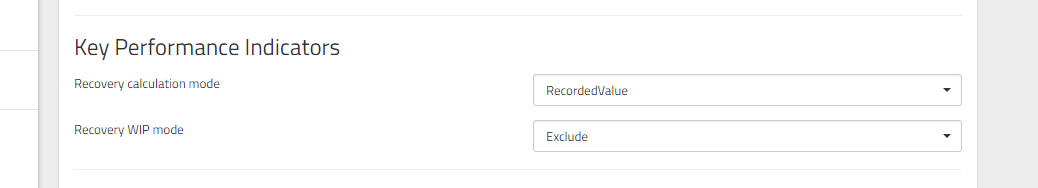In this article, I will explain how the financial values in Clocktimizer are calculated or displayed.

Information imported directly from a firm's data system:

• Recorded Value: often referred to as either recorded value or worked value
• Recorded Rate: imported if provided, otherwise a calculation will be made using the recorded value and the time recorded
• Billed value: what is billed to the client
• Billed rate: imported if provided, otherwise a calculation will be made using the billed value and the time recorded
• Billed value + Work in progress
• Work in progress
• Cost base value:  imported if provided, otherwise a calculation will be made using the cost base rate and the time recorded
• Cost base rate
• Standard value: imported if provided, otherwise a calculation will be made using the standard rate and the time recorded
• Standard rate (or Rack Rate)

Information calculated based on other values:

• Profitability: calculated by subtracting cost base value from billed value, then dividing by billed value. i.e. = (billed value - cost base value) / billed value
• Writeoff: calculated by subtracting work in progress and billed value from recorded value. i.e. = recorded value - billed value - Work in progress
• Blended rate: calculated by dividing the recorded value by hours
• Leverage: calculated by dividing the total hours by partner hours (as defined in the title to level grouping).

• Client count
• Matter count
• Timekeeper count
• Timecard count.

Recovery or Realization:

Recovery can be calculated in 4 different ways and can be configured by the user. The options are:

• Standard recovery, either:
• including Work in progress or,
• excluding Work in progress
• Recorded recovery, either:
• including Work in progress or,
• excluding Work in progress

You can check what type of calculation Clocktimizer is using by going to the Drilldown, selecting the Expanded visualization, and adding "Recovery" in the Display menu. Hovering over the "i" icon will show you the calculation.This can be configured by a user with Administrator permissions by going to Admin in the left menu and clicking Settings, under Install. This option is under the header "Key Performance Indicators". Here you can set to use Standard recovery, Recorded recovery, and if you would like to include Work in progress. The "Auto" calculation mode will use Standard recovery, if unavailable it will use Recorded recovery.